# Build It Up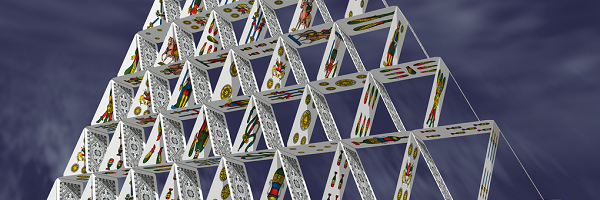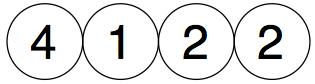We then add in pairs and place the totals above them :-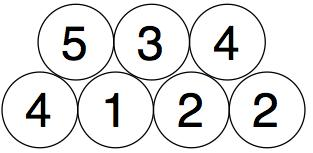And we then add in pairs the new numbers we just got.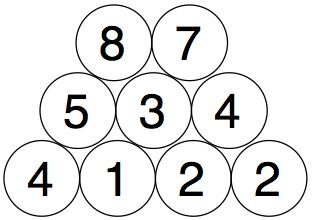We do the same with those two numbers to get our final number.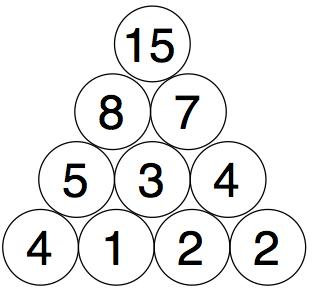You need to find four starting numbers to place at the bottom so that when you get to the top it's 15 each time.

Try to find as many starting four numbers as you can.

Think about good ways to do this. Maybe use a system!

Change things again

What little change can you make to the rules to explore again?

What new ideas can you think of to make this different? Explore those!

Here is a brand new variation that has lots and lots within it to explore  No longer 4 numbers at the bottom,  but 7 and they are 1, 2, 4, 8, 16, 32, 64   build it up in the same way as before and when you've reached the top EXPLORE and CREATE other versions.

### Problem

This is impossible!

### Solution Help

Work from the top to the bottom (start with 15, find two numbers that add up to 15. e.g 10+5)
Then carry on descending until you have your SUPER SOLUTION!!!
We really hope we helped!

### no its not

HOW is this impossible?

### Math

Its not impossible you just need to have two 1s and two other numbers 1-10

### math

true this is impossible

### Keep trying!

What have you done so far? It would be good to know what you have tried so we can try to help.

### build it up

We started at the top and worked down and you can only use 1 at the bottom.
15
96
542
2311 That is our method.

### Well done, Elliott and Toby!

Well done, Elliott and Toby! Can you find any other solutions with 15 at the top?

### How many possible combinations are there?

I have 4 so far- how many ways can you make 15

### That's a really good number of solutions

That's a really good number to find. You might like to look at other numbers at the top instead of 15.

### There are an infinite number of solutions

Say the numbers on the bottom row are a, b, c, and d respectively. The numbers on the second row will thus be a + b, b + c, and c + d. The numbers on the third row are a + 2b + c and b + 2c + d, and finally, the numbers on the top row are a + 3b + 3c + d. Thus, all one needs to do is to find nonzero solutions to the equation a + 3b + 3c + d = 15, of which there are infinitely many solutions.

### It would be good to see the first few

It would be good to see the first few of the"infinitely many" so we can get an idea as to why there re so many. Thanks.

### Some Examples

I've used "Reveal" here because this solution uses Mathematics that many under 14 year olds will probably not have come across and may find off-putting.

For example, a = 1, b = 1, c = 1, and d = 8 a = 0.1, b = 0.2, c = 0.3, and d = 13.4 a = 10/3, b = 16/7, c = 23/21, and d = 32/21 a = -1/5, b = -1/30, c = 1/10, and d = 15 a = -Sin(π/17)^2, b = 16 Cos(π/5)^2 /3, c = 16 Sin(π/5)^2 /3, -Cos(π/17)^2 a = 5i + 7, b = 7i - 8, c = -8i + 3, and d = -2i + 8 and so on...

### Restricting to positive integers

If we restrict this problem to positive integers, this becomes more interesting, because a + 3b + 3c + d = 15 now only has a finite number of solutions: a = 1, b = 1, c = 3, d = 2 a = 2, b = 1, c = 3, d = 1 a = 1, b = 2, c = 2, d = 2 a = 2, b = 2, c = 2, d = 1 a = 1, b = 3, c = 1, d = 2 a = 2, b = 3, c = 1, d = 1 a = 1, b = 1, c = 2, d = 5 a = 5, b = 1, c = 2, d = 1 a = 1, b = 2, c = 1, d = 5 a = 5, b = 2, c = 1, d = 1 a = 2, b = 1, c = 2, d = 4 a = 4, b = 1, c = 2, d = 2 a = 2, b = 2, c = 1, d = 4 a = 4, b = 2, c = 1, d = 2 a = 3, b = 1, c = 2, d = 3 a = 3, b = 1, c = 2, d = 3 a = 3, b = 2, c = 1, d = 3 a = 3, b = 2, c = 1, d = 3 a = 1, b = 1, c = 1, d = 8 a = 8, b = 1, c = 1, d = 1 a = 2, b = 1, c = 1, d = 7 a = 7, b = 1, c = 1, d = 2 a = 3, b = 1, c = 1, d = 6 a = 6, b = 1, c = 1, d = 3 a = 4, b = 1, c = 1, d = 5 a = 5, b = 1, c = 1, d = 4

I've used reveal here so that those who want to work on it on their own will not instantly see a solution without pressing "reveal".

### Great thanks for that, you've

Great thanks for that, you've done some good work here.

### We loved this!

We loved this!
Stingrays class spent twenty minutes in competition with each other to create pyramids that added to fifteen. We double checked them all and we found 18 solutions!!
A great puzzle we really enjoyed.

### Good to hear you liked it, it

Good to hear you liked it, it may inspire others.

### We think there are more than 18

We started at the top and found out that is the smallest left hand number in row 2 (as you can't have 1 in any row except the bottom)
Increasing by one eactime we think there are 12 and then each of those can be done in reverse (read right to left) to make 24 total

### Our solution

We came up with 22 solutions and we found them by starting from the top. We switched some numbers around to create more solutions and we worked out that if the numbers in the middle add to 2 the numbers on the outside must add to 9. Also, the numbers beneath the 15 cannot be 1, 2 or 3. The formula is a+3b+3c+d equals 15. You can swap the numbers in the middle and the numbers on the edge of the bottom row.

I've used "Reveal" here because this solution uses Mathematics that many under 14 year olds will probably not have come across and may find off-putting.

### Whoops

We just found another one

### can you use negative numbers

if you can use negative numbers please say because if you can it will open up a lot of new answers

### It's for you to decide about

It's for you to decide about the use of negative numbers.

### So it's a matter of finding 4

So it's a matter of finding 4 numbers, placing them in a row. Adding the first two together and putting the total above (probably above the space between your first two numbers). Doing the same with the 2nd and 3rd numbers and putting the total above (like in the first pair). Similarly for the last pair. Then you look at your new 3 numbers and add the first pair and put the total above, then similarly with the 2nd pair. You then look at your latest two answers and add them up and get a final answer and put that at the top. The exploration is about working out how to choose 4 starting numbers that will give you a final 15 at the top!

### awesome

simple to learn but hard to master. There are a few ways to make 15 that I worked out but once I thought that there was no more ways to make 15 I cheated a bit because I put 15 at the top and worked from there. If you are struggling 3213

### Complicated Addition or Subtraction - Making a Pyramid

Many of us started at the top and worked our way down by breaking down the numbers until we got to the bottom row. There are LOTs of solutions for example

15
9 6
5 4 2
2 3 1 1

### My method

work from the top down

15
7 8
3 4 4
2 1 3 1

15
9 6
6 3 3
4 2 1 2

### A Method That May Not Be Helpful

One of the ways to do this is, of course, work from the top to the bottom but some people may class that as cheating so I have NOT come up with another method for all honestiores around here. You may not find this at all helpful but for example... (It's just something I wanted to do.) 15 can be divided by 4 but in decimal numbers, you can use decimals in your sum if you would like to, that is a harder option for people who want a challenge so let's say a = 0.1, b = 0.9, c = 1.9 and that will add up to 2.9, take that away from 15 and it will equal 12.1. So d= 12.1. OR... You can use four numbers at the bottom and multiply them, but only multiply the ones at the bottom. The rest you can either multiply or add but I think multiplying the next ones are too hard. If you find multiplying four numbers too hard try three, and if still difficult try two and work your way up to three numbers then four. I hope you like these ways of making things more difficult. THANKS

### Whole number solution

We used only whole numbers. You cannot have an infinite number of solutions. The numbers 1, 2 and 3 cannot appear in the second row otherwise 1 will appear in the third row and you can't have 0 in the bottom row. 'There always has to be a 1 in the bottom row. If you have 4122 you can't count 2214 as it is just the same numbers but backwards. We found 11 solutions: 4122, 2131, 1118, 2221, 5211, 4115, 1132, 7112, 3116, 4212 and 3123.

### There is twelve

Ther is twelve ways to make it

### What age is this appropriate for?

will a year 1 student be able to solve this, i mean finding an alternate combination to get 15 on the top

### There will probably be some

There will probably be some year 1's who will manage this, particularly if they have some physical numbers to move around.

### Maths Build It Up Problem

Our class found 23 solutions to the problem! We worked hard to swap numbers around and we used a lot of trial and error. The children did not start at the top and tackled this problem bravely and with perseverance. I am very proud :)

### Only certain numbers work.

1,2 and 3 don't work at the third line/column up because for 1 a number + a number is higher than 1 in the second line up. As a result, we cannot make 1, 2 (it would be 1+1) or 3 (2+1 or 1+2). We found 4 answers so far: 15 4 11 2 2 9 1 1 1 8 15 5 10 2 3 7 1 1 2 5 15 6 9 3 3 6 2 1 2 4 15 7 8 4 3 5 3 1 2 3

### BUILD UP

This is a challenge of mathematics. We have tried to start from the bottom but it was difficult so we went to the top.We tried to like split 15 in different numbers then we split and split util we get to the bottom. Here are some examples we have found: 5+10=15 15 5 10 2 3 7 1 1 2 5 15 6 9 2 4 5 1 1 3 2

### Number of solutions

Hello,

How many solutions did you find when you used different digits on the bottom row? We currently have 9 different sets of numbers on the bottom row!

### Build it up

Can you put ten in?

### maths

if you take four numbers like 4,1,2,2 you can make lots of build up

### Yes Ricardo it's about being

Yes Ricardo it's about being creative.

### Build it up

Our class found 12 solutions

15 15 15 15 15 15 15 15 15 15 15 6 9 1 1 4 7 8 6 9 7 8 5 10 6 9 6 9 8 7 10 5 8 7 3 3 6 9 2 2 3 4 4 2 4 5 3 4 4 3 2 8 3 3 6 4 2 7 6 2 5 7 3 2 5 3 4 2 1 2 4 8 1 1 1 1 2 2 2 1 1 3 2 2 1 3 1 2 1 1 7 1 2 1 5 3 1 1 6 5 1 1 4 5 2 1 1 4 1 2 2 15 8 7 5 3 4 3 2 1 3

### We're proud of ourselves!

Hello World! :)

We are the students of Room 11. A Year 4 classroom in Waterlea Primary School, in Auckland, New Zealand. Early on in the challenge, we found that starting from the top made it easier. We then tried many combinations of numbers and had 40 different solutions! Our teacher showed us that we had a few solutions that had doubled up, so we removed them. Then, we double-checked our answers and found that some were incorrect! Finally, we ended 22 different solutions. We are all very proud of the effort we put in!

### I know it!!!!

On the bottom row is A B C D.

B and C can only be 1, 2 or 3 If one is three then the other must be one. you multiply your two chosen numbers by three the subtract it from 15. The answer is the sum of A and D. All you have to do is choose the two numbers, A and D

### I know it !!!!

Thanks for your very creative solution - I like it a lot! I have hidden your words so that they only will be revealed upon a "click". This is so that folk can avoid seeing it straight away and so "spoil" their own creativity. Thanks